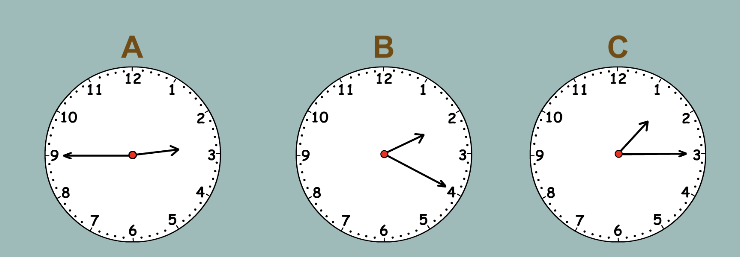ClockWork 10
Set 10 (Recognize and resolve expressions like, "How many minutes are in one and a half hours...", when shown an analog clock.)
From Mr. Anker Tests1. How many hours are equal to sixty minutes?
1 point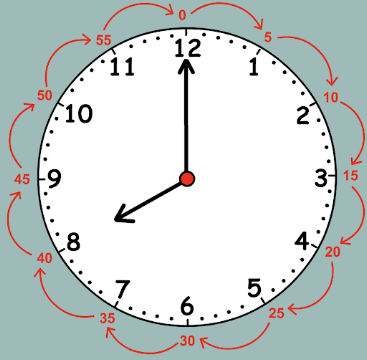2. How many hours are equal to 30 minutes? *
1 point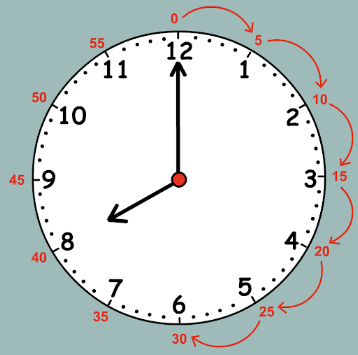3. How many minutes are there in 10 hours? *
1 point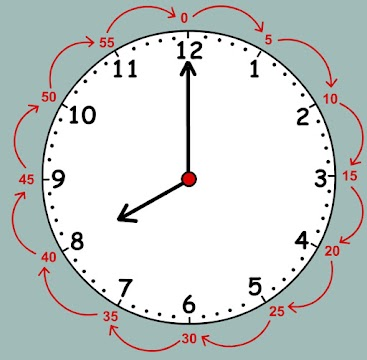4. How many minutes are there in 1/4 hours? *
1 point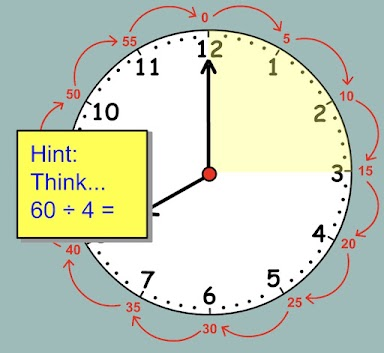5. How many minutes are there in one and a half hours? *
1 point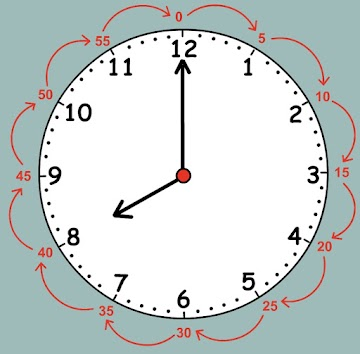6. Which of the clocks below shows a time that is two and a half hours after six? *
1 point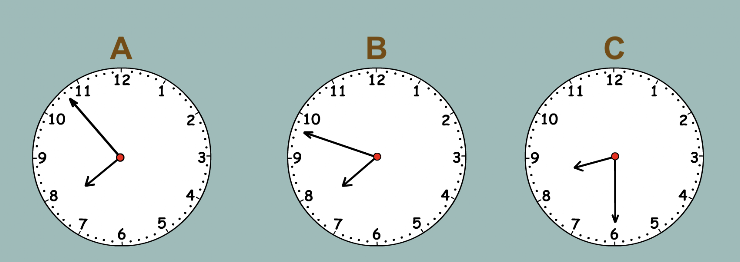7. Which of the clocks below shows a time that is exactly 120 minutes after eight? *
1 point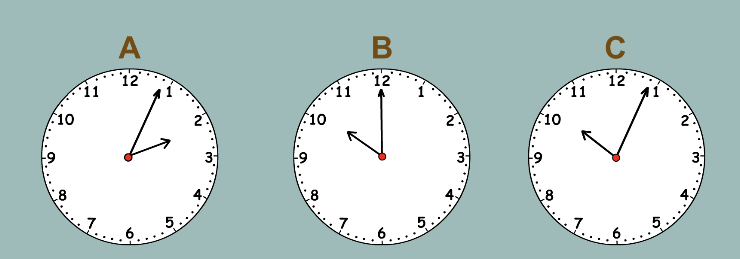8. Which of the clocks below shows the time, "five 'til ten"? *
1 point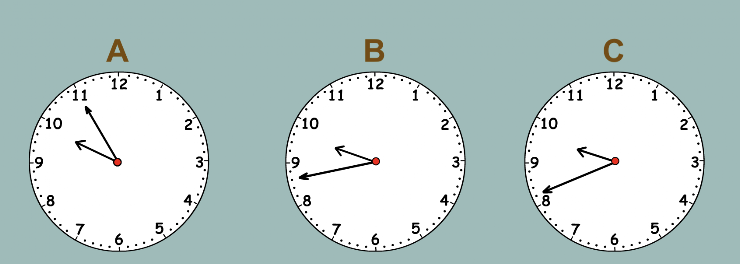9. Which of the clocks below shows a time that is 90 minutes before 2:00? *
1 point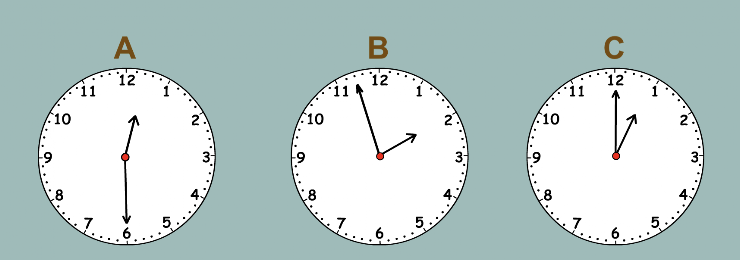10. Which of the clocks below shows a time that is 75 minutes past noon? *
1 point